# D. Vulcanov - On Cosmologies with non-Minimally Coupled Scalar Field and the "Reverse Engineering Method"

Sep. 11, 2011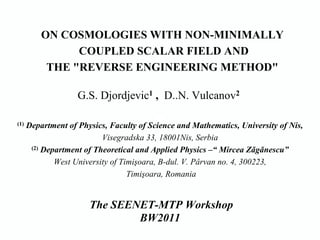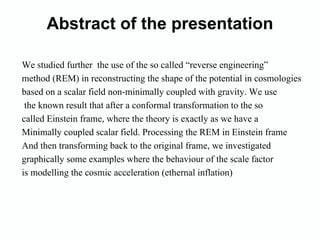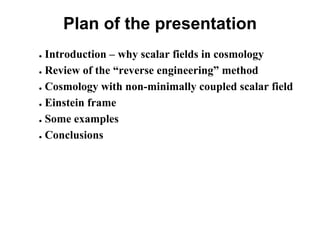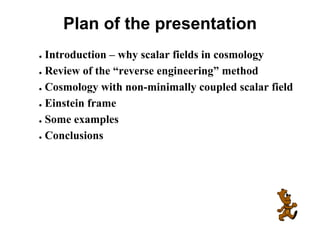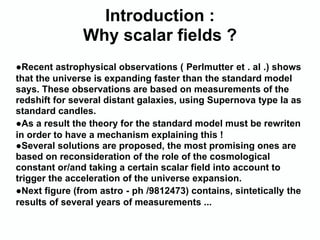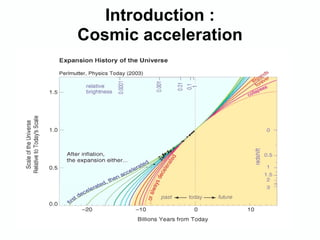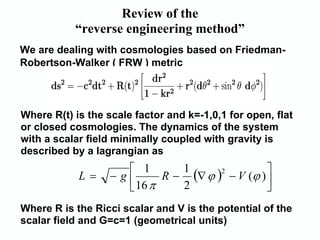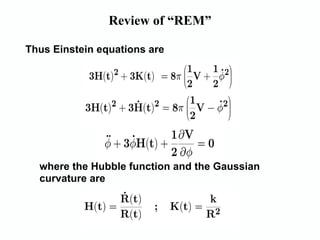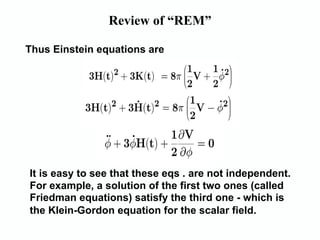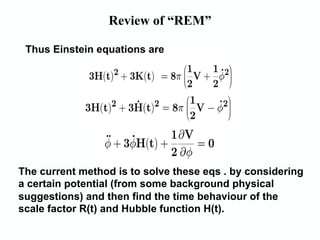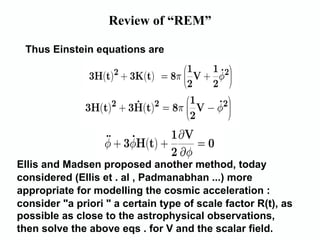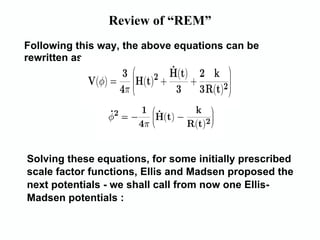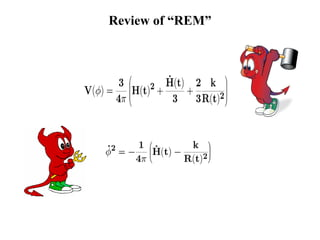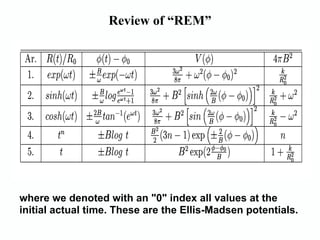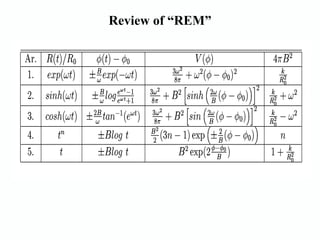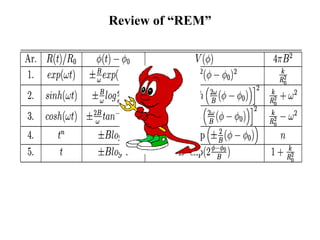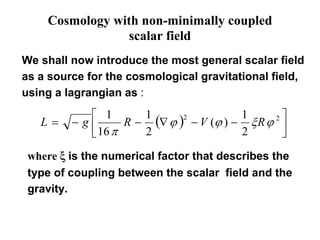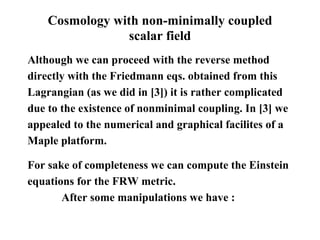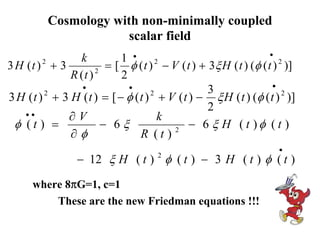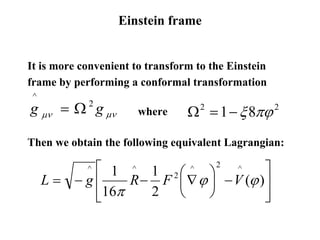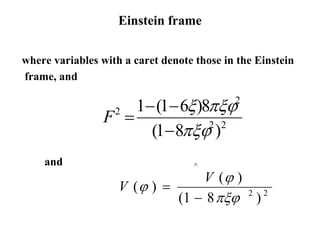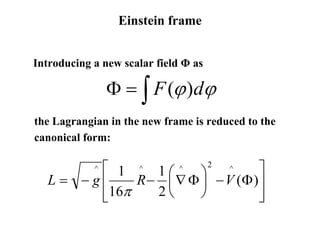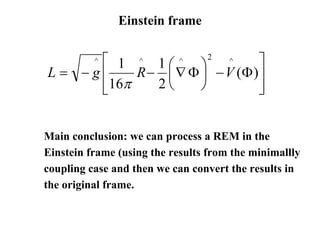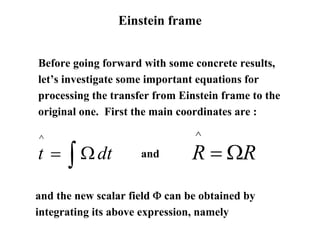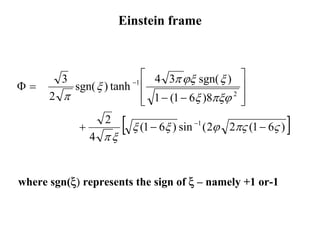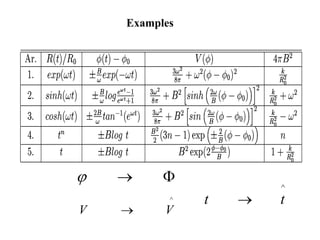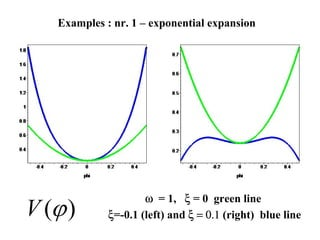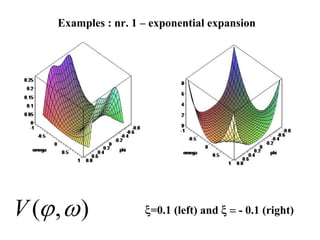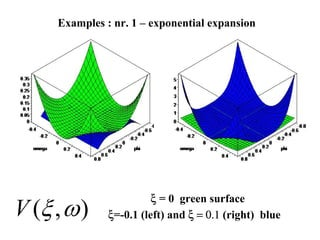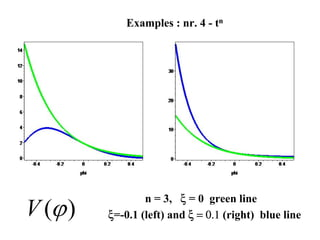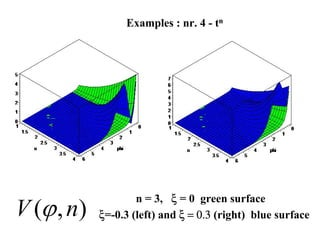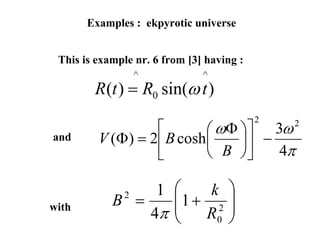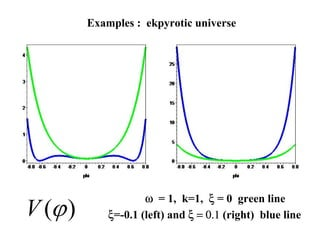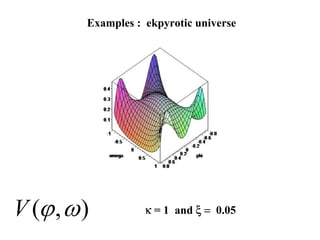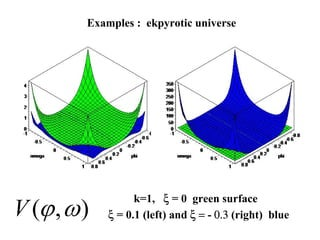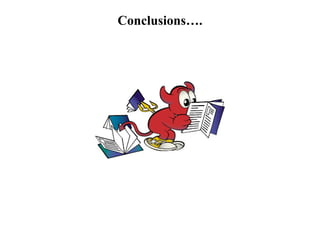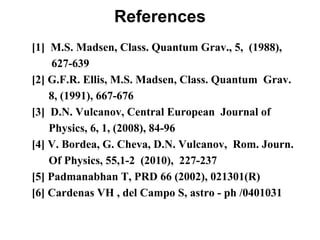1 of 39

### D. Vulcanov - On Cosmologies with non-Minimally Coupled Scalar Field and the "Reverse Engineering Method"

• 1. ON COSMOLOGIES WITH NON-MINIMALLY COUPLED SCALAR FIELD AND THE "REVERSE ENGINEERING METHOD" G.S. Djordjevic1 , D..N. Vulcanov2 (1) Department of Physics, Faculty of Science and Mathematics, University of Nis, Visegradska 33, 18001Nis, Serbia (2) Department of Theoretical and Applied Physics –“ Mircea Zǎgǎnescu” West University of Timişoara, B-dul. V. Pârvan no. 4, 300223, Timişoara, Romania The SEENET-MTP Workshop BW2011
• 2. Abstract of the presentation We studied further the use of the so called “reverse engineering” method (REM) in reconstructing the shape of the potential in cosmologies based on a scalar field non-minimally coupled with gravity. We use the known result that after a conformal transformation to the so called Einstein frame, where the theory is exactly as we have a Minimally coupled scalar field. Processing the REM in Einstein frame And then transforming back to the original frame, we investigated graphically some examples where the behaviour of the scale factor is modelling the cosmic acceleration (ethernal inflation)
• 3. Plan of the presentation ● Introduction – why scalar fields in cosmology ● Review of the “reverse engineering” method ● Cosmology with non-minimally coupled scalar field ● Einstein frame ● Some examples ● Conclusions
• 4. Plan of the presentation ● Introduction – why scalar fields in cosmology ● Review of the “reverse engineering” method ● Cosmology with non-minimally coupled scalar field ● Einstein frame ● Some examples ● Conclusions
• 5. Introduction : Why scalar fields ? ●Recent astrophysical observations ( Perlmutter et . al .) shows that the universe is expanding faster than the standard model says. These observations are based on measurements of the redshift for several distant galaxies, using Supernova type Ia as standard candles. ●As a result the theory for the standard model must be rewriten in order to have a mechanism explaining this ! ●Several solutions are proposed, the most promising ones are based on reconsideration of the role of the cosmological constant or/and taking a certain scalar field into account to trigger the acceleration of the universe expansion. ●Next figure (from astro - ph /9812473) contains, sintetically the results of several years of measurements ...
• 6. Introduction : Cosmic acceleration
• 7. Review of the “reverse engineering method” We are dealing with cosmologies based on Friedman- Robertson-Walker ( FRW ) metric Where R(t) is the scale factor and k=-1,0,1 for open, flat or closed cosmologies. The dynamics of the system with a scalar field minimally coupled with gravity is described by a lagrangian as ⎡ 1 ⎤ R − (∇ ϕ ) − V (ϕ ) ⎥ 1 L= −g⎢ 2 ⎣ 16 π 2 ⎦ Where R is the Ricci scalar and V is the potential of the scalar field and G=c=1 (geometrical units)
• 8. Review of “REM” Thus Einstein equations are where the Hubble function and the Gaussian curvature are
• 9. Review of “REM” Thus Einstein equations are It is easy to see that these eqs . are not independent. For example, a solution of the first two ones (called Friedman equations) satisfy the third one - which is the Klein-Gordon equation for the scalar field.
• 10. Review of “REM” Thus Einstein equations are The current method is to solve these eqs . by considering a certain potential (from some background physical suggestions) and then find the time behaviour of the scale factor R(t) and Hubble function H(t).
• 11. Review of “REM” Thus Einstein equations are Ellis and Madsen proposed another method, today considered (Ellis et . al , Padmanabhan ...) more appropriate for modelling the cosmic acceleration : consider "a priori " a certain type of scale factor R(t), as possible as close to the astrophysical observations, then solve the above eqs . for V and the scalar field.
• 12. Review of “REM” Following this way, the above equations can be rewritten as Solving these equations, for some initially prescribed scale factor functions, Ellis and Madsen proposed the next potentials - we shall call from now one Ellis- Madsen potentials :
• 13. Review of “REM”
• 14. Review of “REM” where we denoted with an "0" index all values at the initial actual time. These are the Ellis-Madsen potentials.
• 15. Review of “REM”
• 16. Review of “REM”
• 17. Cosmology with non-minimally coupled scalar field We shall now introduce the most general scalar field as a source for the cosmological gravitational field, using a lagrangian as : ⎡ 1 ⎤ R − (∇ ϕ ) − V (ϕ ) − ξ R ϕ 2 ⎥ 1 1 L= −g⎢ 2 ⎣ 16 π 2 2 ⎦ where ξ is the numerical factor that describes the type of coupling between the scalar field and the gravity.
• 18. Cosmology with non-minimally coupled scalar field Although we can proceed with the reverse method directly with the Friedmann eqs. obtained from this Lagrangian (as we did in ) it is rather complicated due to the existence of nonminimal coupling. In  we appealed to the numerical and graphical facilites of a Maple platform. For sake of completeness we can compute the Einstein equations for the FRW metric. After some manipulations we have :
• 19. Cosmology with non-minimally coupled scalar field • k 1 • 2 3 H (t ) 2 + 3 = [ φ ( t ) − V ( t ) + 3ξ H ( t ) (φ ( t ) 2 )] R (t ) 2 2 • • • 3 3 H ( t ) 2 + 3 H ( t ) = [ − φ ( t ) 2 + V ( t ) − ξ H ( t ) (φ ( t ) 2 )] 2 •• ∂V k φ (t ) = − 6ξ − 6 ξ H ( t )φ ( t ) ∂φ R (t ) 2 • − 12 ξ H ( t ) φ ( t ) − 3 H ( t ) φ ( t ) 2 where 8πG=1, c=1 These are the new Friedman equations !!!
• 20. Einstein frame It is more convenient to transform to the Einstein frame by performing a conformal transformation ^ g µν = Ω 2 g µν where Ω 2 = 1 − ξ 8πϕ 2 Then we obtain the following equivalent Lagrangian: ⎡ 1 ^ 1 2 ⎛ ^ ⎞2 ^ ^ ⎤ L= −g⎢ R − F ⎜ ∇ ϕ ⎟ − V (ϕ ) ⎥ ⎢16π ⎣ 2 ⎝ ⎠ ⎥ ⎦
• 21. Einstein frame where variables with a caret denote those in the Einstein frame, and 1− (1− 6ξ )8πξϕ 2 F = 2 (1− 8πξϕ )2 2 and ^ V (ϕ ) V (ϕ ) = (1 − 8 πξϕ ) 2 2
• 22. Einstein frame Introducing a new scalar field Φ as Φ = ∫ F (ϕ )dϕ the Lagrangian in the new frame is reduced to the canonical form: ⎡ 1 ^ 1 ⎛ ^ ⎞2 ^ ^ ⎤ L= −g⎢ R − ⎜ ∇ Φ ⎟ − V (Φ ) ⎥ ⎢16π ⎣ 2⎝ ⎠ ⎥ ⎦
• 23. Einstein frame ⎡ 1 ^ 1 ⎛ ^ ⎞2 ^ ^ ⎤ L= −g⎢ R − ⎜ ∇ Φ ⎟ − V (Φ ) ⎥ ⎢16π ⎣ 2⎝ ⎠ ⎥ ⎦ Main conclusion: we can process a REM in the Einstein frame (using the results from the minimallly coupling case and then we can convert the results in the original frame.
• 24. Einstein frame Before going forward with some concrete results, let’s investigate some important equations for processing the transfer from Einstein frame to the original one. First the main coordinates are : ^ ^ t= ∫ Ω dt and R = ΩR and the new scalar field Φ can be obtained by integrating its above expression, namely
• 25. Einstein frame 3 ⎡ 4 3π ϕξ sgn( ξ ) ⎤ Φ= sgn( ξ ) tanh ⎢ −1 ⎥ 2 π ⎢ 1 − (1 − 6ξ )8πξϕ 2 ⎣ ⎥ ⎦ + 4 2 πξ [ ξ (1 − 6ξ ) sin −1 ( 2ϕ 2πς (1 − 6ς ) ] where sgn(ξ) represents the sign of ξ – namely +1 or-1
• 26. Examples ϕ → Φ ^ ^ t → t V → V
• 27. Examples : nr. 1 – exponential expansion V (ϕ ) ω = 1, ξ = 0 green line ξ=-0.1 (left) and ξ = 0.1 (right) blue line
• 28. Examples : nr. 1 – exponential expansion V (ϕ , ω ) ξ=0.1 (left) and ξ = - 0.1 (right)
• 29. Examples : nr. 1 – exponential expansion V (ξ , ω ) ξ = 0 green surface ξ=-0.1 (left) and ξ = 0.1 (right) blue
• 30. Examples : nr. 4 - tn V (ϕ ) n = 3, ξ = 0 green line ξ=-0.1 (left) and ξ = 0.1 (right) blue line
• 31. Examples : nr. 4 - tn V (ϕ , n) n = 3, ξ = 0 green surface ξ=-0.3 (left) and ξ = 0.3 (right) blue surface
• 32. Examples : ekpyrotic universe This is example nr. 6 from  having : ^ ^ R (t ) = R0 sin(ω t ) 2 ⎡ ⎛ ωΦ ⎞⎤ 3ω 2 and V (Φ ) = 2 ⎢ B cosh⎜ ⎟⎥ − ⎣ ⎝ B ⎠⎦ 4π 1 ⎛ k ⎞ B = 2 ⎜1 + 2 ⎟ ⎜ with 4π ⎝ R0 ⎟ ⎠
• 33. Examples : ekpyrotic universe V (ϕ ) ω = 1, k=1, ξ = 0 green line ξ=-0.1 (left) and ξ = 0.1 (right) blue line
• 34. Examples : ekpyrotic universe V (ϕ , ω ) κ = 1 and ξ = 0.05
• 35. Examples : ekpyrotic universe V (ϕ , ω ) k=1, ξ = 0 green surface ξ = 0.1 (left) and ξ = - 0.3 (right) blue
• 36. Conclusions….
• 37. Conclusions….
• 38. References  M.S. Madsen, Class. Quantum Grav., 5, (1988), 627-639  G.F.R. Ellis, M.S. Madsen, Class. Quantum Grav. 8, (1991), 667-676  D.N. Vulcanov, Central European Journal of Physics, 6, 1, (2008), 84-96  V. Bordea, G. Cheva, D.N. Vulcanov, Rom. Journ. Of Physics, 55,1-2 (2010), 227-237  Padmanabhan T, PRD 66 (2002), 021301(R)  Cardenas VH , del Campo S, astro - ph /0401031
• 39. The end !!! Thank you for your attention !